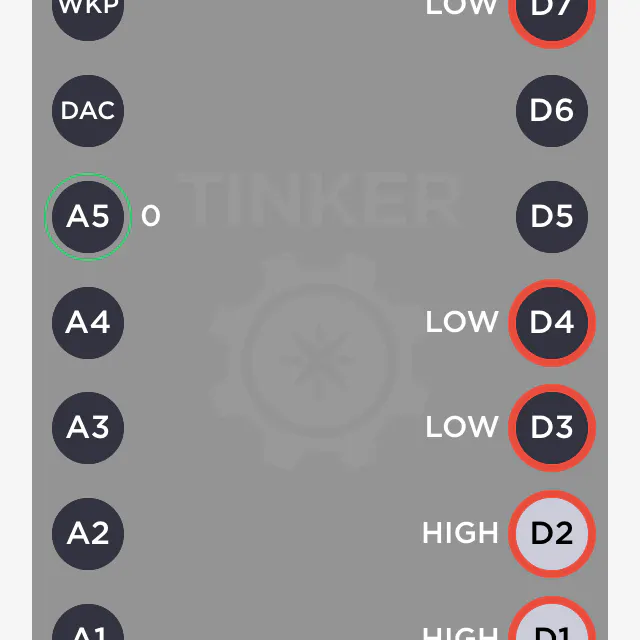# 7-Segment Clock with Arduino Nano + DS3231 + LDR

Another clock project with 1, 2" matrix display.

BeginnerFull instructions provided1.5 hours15,341

## Things used in this project

### Hardware componentsArduino Nano R3 works well with Arduino Uno or Elegoo Uno
×1
 Adafruit 7-Segment Backpack - 1.2" Tall Digits - 1.2" Tall Digits in my case (optional if you buy the bundle)
×1
 Adafruit 1.2 4-Digit 7-Segment Display w/I2C Backpack - Green
×1
×1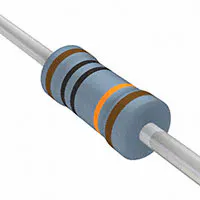Resistor 100k ohm
×1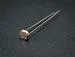Photo resistor
×1×1Jumper wires (generic) 10-14 pieces
×1
 Coin Cell Battery CR1220
×1

### Software apps and online servicesArduino IDE

## Schematics

### MyClock v1.1

LDR path corrected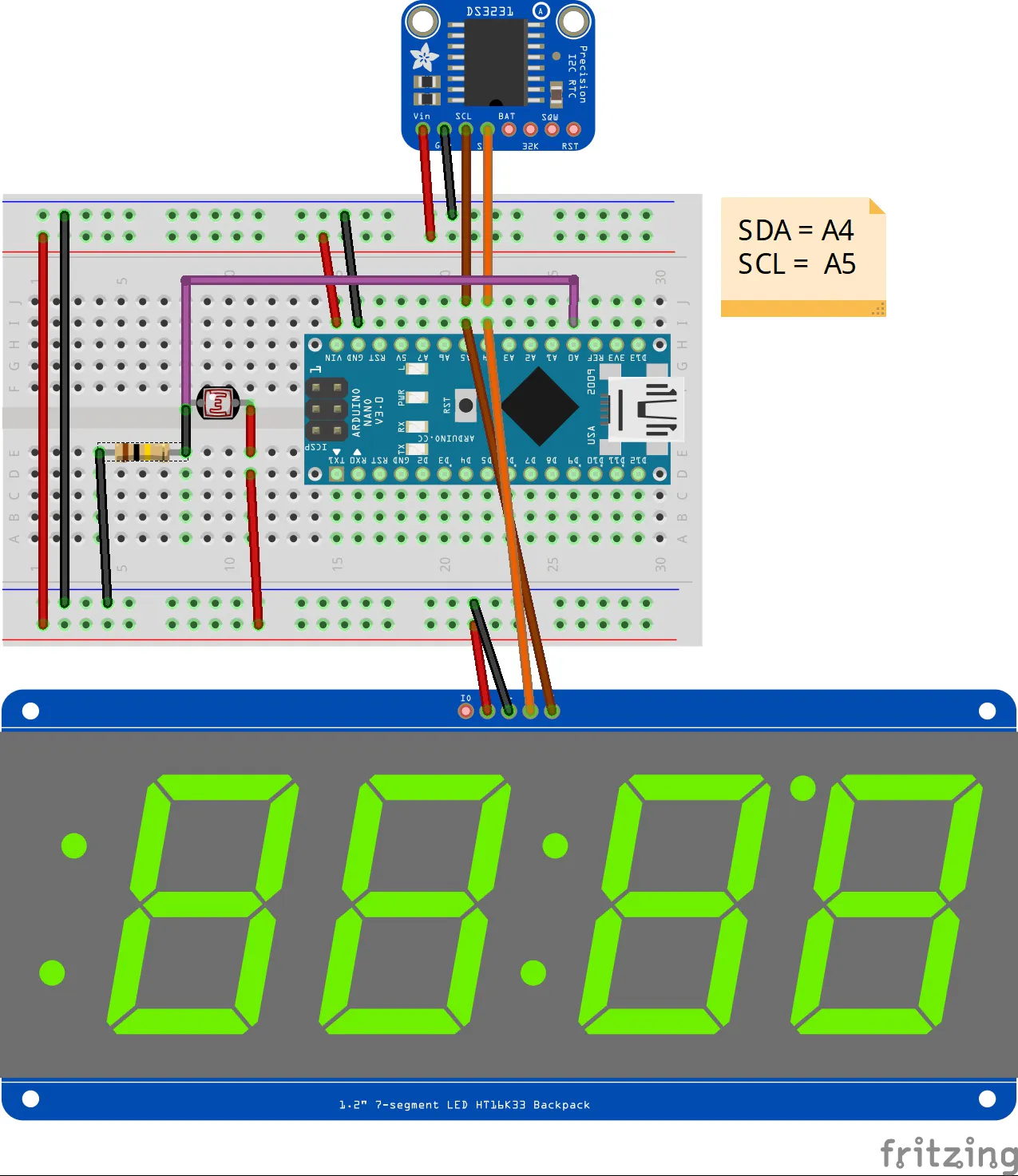### MyClock v1.0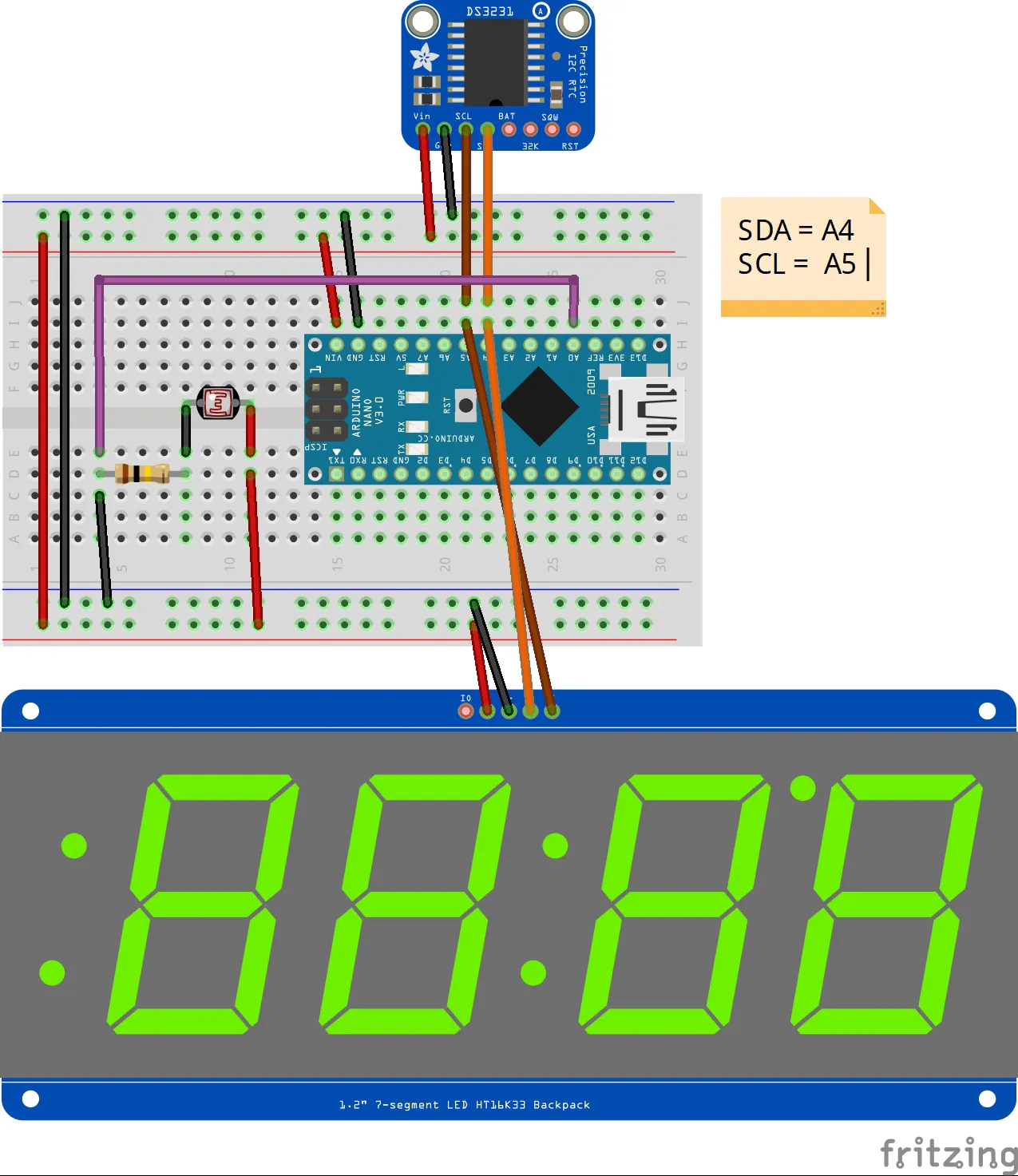## Code

### MyClock v1.0

C/C++
```// MyClock v1.0
// Ingo Lohs
// Hardware: tested with Arduino Uno and Arduino Nano (ATmega328P [Old Bootloader]) by using Arduino IDE v1.8.8

/* Theory for the Matrix ht16k33
The easiest is to just call print - just like you do with Serial
print(variable,HEX) - this will print a hexidecimal number, from 0000 up to FFFF
print(variable,DEC) or
print(variable) - this will print a decimal integer, from 0000 up to 9999

If you need more control, you can call writeDigitNum(location, number) - this will write the number (0-9) to a single location.
Location #0 is all the way to the left, location #2 is the colon dots so you probably want to skip it, location #4 is all the way to the right.

To control the colon and decimal points, use the writeDigitRaw(location, bitmap) function.
(Note that both dots of the center colon are wired together internal to the display, so it is not possible to address them separately.)

Specify 2 for the location and the bits are mapped as follows:
0x02 - center colon (both dots)
0x04 - left colon - lower dot
0x08 - left colon - upper dot
0x10 - decimal point

If you want a decimal point, call writeDigitNum(location, number, true) which will paint the decimal point.
To draw the colon, use drawColon(true or false)

If you want full control of the segments in all digits, you can call writeDigitRaw(location,bitmask) to draw a raw 8-bit mask (as stored in a uint8_t) to any location.

All the drawing routines only change the display memory kept by the Arduino. Don't forget to call writeDisplay() after drawing to 'save' the memory out to the matrix via I2C.

*/

#include <Wire.h> // Enable this line if using Arduino Uno, Mega, etc.

int ldr_sensor = A0;            // LDR Photoresistor with 100k Ohm to GND, other leg 5V
int ldr_value = 0;              // LDR value - var store value
int brightness_matrix;          // LED Matrix brightness - var store value
int threshold_brightness = 400; // Threshold
int delay_matrix_time = 20;     // how long display time (20 sec)
int delay_matrix_temp = 2000;   // how long display temp (2 sec)
int blinky_dot = 2;             // blinks nothing - on the left side 2 single dots

byte decToBcd(byte val){
return ( (val/10*16) + (val%10) );
}

byte bcdToDec(byte val){
return ( (val/16*10) + (val%16) );
}

void setup() {
Serial.begin(9600);
Wire.begin();
matrix.begin(0x70);
}

void loop() {
lightBrightness(); // Matrix-Brightness Justierung
displayTime();     // display the real-time clock data
displayTemp();     // display the temperature
}

void lightBrightness() {
// setBrightness(brighness) - will let you change the overall brightness of the entire display. 0 is least bright, 15 is brightest and is what is initialized by the display when you start
Serial.print("LDR: ");
Serial.println(ldr_value);
if (ldr_value <= threshold_brightness) { // measurement of brightness vs Threshold
brightness_matrix = 0; // bright at daylight
}
else {
brightness_matrix = 15; // dark at night
}
}

}

void displayTime(){
matrix.setBrightness(brightness_matrix);
matrix.clear();

// how long display time on matrix in seconds
for (uint16_t i = 0; i < delay_matrix_time; i++) {
byte second, minute, hour, dayOfWeek, dayOfMonth, month, year;
getDateDs3231(&second, &minute, &hour, &dayOfWeek, &dayOfMonth, &month, &year);
byte se = second;
byte mi = minute;
byte ho = hour;
byte we = dayOfWeek;
byte dm = dayOfMonth;
byte mo = month;
byte ye = year;

// změna času na letní - poslední neděle v březnu ve 2 hodiny
// >> Change to summer time - last Sunday in March at 2 o'clock
if ((dayOfWeek == 7) && (dayOfMonth >= 25)&& (month == 3) && (hour == 2)) {
// nastavení hodin na 3 hodinu
// set the clock to 3 hours
setDS3231time(se,mi,3,we,dm,mo,ye);
}

// změna času na zimní
// >> Change to winter time
if ((dayOfWeek == 7) && (dayOfMonth >= 25) && (month == 10) && (hour == 1)&& (year != 1)) {

// rok použit jako indikace, že bylo léto
// year used as an indication that it was summer
setDS3231time(se,mi,ho,we,dm,mo,1);
}

if ((dayOfWeek == 7) && (dayOfMonth >= 25) && (month == 10) && (hour == 3) &&(year == 1)) {
// nastavení hodin na 2 hodinu a příznak na 0
// set the clock to 2 hours and flag year to 0
setDS3231time(se,mi,2,we,dm,mo,0);
}

/*
Specify 2 for the location and the bits are mapped as follows:
0x02 - center colon (both dots)
0x04 - left colon - lower dot
0x08 - left colon - upper dot
0x10 - decimal point
*/

/* location #2 controls also the left 2 single points like this (=blinky_dot):
4 = blinks top left (single upper dot)
5 = blinks top left (single upper dot)
6 = blinks top left (single upper dot) + both Colon in mid
7 = blinks top left (single upper dot) + both Colon in mid
8 = blinks bottom left (single lower dot)
9 = blinks bottom left (single lower dot)
10 = blinks bottom left (single lower dot) + both Colon in mid
11 = blinks bottom left (single lower dot) + both Colon in mid
12 = blinks both Colon (both single dots left) without both Colon in mid
*/

// format hours: _0:mm
if (hour > 9) {
matrix.writeDigitNum(0, (hour / 10), false);
matrix.writeDigitNum(1, (hour % 10), false);    // this will write the number (0-9) to a single location by using modulo https://www.arduino.cc/reference/en/language/structure/arithmetic-operators/modulo/
} else {
matrix.writeDigitNum(1, hour, false);
}

// matrix.drawColon(true);                    // both Colon in mid active: in case you want not blink the dots left you can activate the both Colon here

// give seconds a chance to show at left location dots
if (se <= 30) {
blinky_dot = 10; // blinks bottom left (single lower dot) + both Colon in mid
} else if (se > 30) {
blinky_dot = 6;   // blinks top left (single upper dot) + both Colon in mid
}

matrix.writeDigitNum(3, (minute / 10), false);
matrix.writeDigitNum(4, (minute % 10), false);

matrix.writeDisplay();

// blinky double dots for half seconds
delay(500);
matrix.drawColon(false);
matrix.writeDisplay();
delay(500);
}
}

void displayTemp(){
matrix.setBrightness(brightness_matrix);
matrix.clear();

byte temp = get3231Temp();
int abs_temp = abs(temp);                                           // absolute number of a value

matrix.writeDigitNum(1,(abs_temp % 10), false);                     // position 1, value 9, show decimal)

if (temp < 0)    matrix.writeDigitRaw(0,64);
if (temp <= -10) matrix.writeDigitRaw(2,12);                        // and if the temperature is negative, we plot the minus sign to first place.
if (temp >= 10)  matrix.writeDigitNum(0, (abs_temp/10), false);     // position 0, value 1, show decimal)
if (temp <= -10) matrix.writeDigitNum(0, (abs_temp/10), false);     // position 0, value 1, show decimal)
// matrix.writeDigitRaw(2,0x10);                                    // decimal dot

matrix.writeDigitRaw(3,99);                                         // 99 = "°"
matrix.writeDigitRaw(4,57);                                         // 57 = "C"
matrix.writeDisplay();

// displays temperature values on the serial line
// zobrazí hodnoty teploty na seriove lince
Serial.print("Temperatur in C: ");
Serial.println(get3231Temp());                                      // +/- 3 Grad Celsius

// how long display temp on matrix in seconds
delay(delay_matrix_temp);
}

void setDS3231time(byte second, byte minute, byte hour, byte dayOfWeek, byte dayOfMonth, byte month, byte year){
// set time and date data to DS3231
Wire.write(0);                    // set next input to start at the seconds register
Wire.write(decToBcd(second));     // set seconds
Wire.write(decToBcd(minute));     // set minutes
Wire.write(decToBcd(hour));       // set hours
Wire.write(decToBcd(dayOfWeek));  // set day of week (1=Sunday, 7=Saturday)
Wire.write(decToBcd(dayOfMonth)); // set date (1 to 31)
Wire.write(decToBcd(month));      // set month
Wire.write(decToBcd(year));       // set year (0 to 99)
Wire.endTransmission();
}

void getDateDs3231
(byte *second,
byte *minute,
byte *hour,
byte *dayOfWeek,
byte *dayOfMonth,
byte *month,
byte *year)
{
Wire.write(0);
Wire.endTransmission();
}

float get3231Temp(){
byte tMSB, tLSB;
float temp3231;

Wire.write(0x11);
Wire.endTransmission();

if (Wire.available()) {
tMSB = Wire.read();                 // 2's complement int portion
tLSB = Wire.read();                 // fraction portion
temp3231 = (tMSB & B01111111);      // do 2's math on Tmsb
temp3231 += ( (tLSB >> 6) * 0.25 ); // only care about bits 7 & 8

return temp3231;
}
else {
// oh no, no data!
Serial.println("DS3231 Sensor Error - cant read temperature");
matrix.print(0xBEEF, HEX); // inform the user with BEEF
matrix.writeDisplay();
delay(5000);
}
}
```

### MyClock v1.1

C/C++
Update Summer/Winter-Time
```// MyClock v1.1
// Ingo Lohs
// Hardware: tested with Arduino Uno and Arduino Nano (ATmega328P [Old Bootloader]) by using Arduino IDE v1.8.8

// Change v1.0 > v1.1
// dayOfWeek == 1 anstatt 7 abgeändert in displayTime() zur korrekten Ermittlung des Sonntages für die Zeitumstellung

/* Theory for the Matrix ht16k33
The easiest is to just call print - just like you do with Serial
print(variable,HEX) - this will print a hexidecimal number, from 0000 up to FFFF
print(variable,DEC) or
print(variable) - this will print a decimal integer, from 0000 up to 9999

If you need more control, you can call writeDigitNum(location, number) - this will write the number (0-9) to a single location.
Location #0 is all the way to the left, location #2 is the colon dots so you probably want to skip it, location #4 is all the way to the right.

To control the colon and decimal points, use the writeDigitRaw(location, bitmap) function.
(Note that both dots of the center colon are wired together internal to the display, so it is not possible to address them separately.)

Specify 2 for the location and the bits are mapped as follows:
0x02 - center colon (both dots)
0x04 - left colon - lower dot
0x08 - left colon - upper dot
0x10 - decimal point

If you want a decimal point, call writeDigitNum(location, number, true) which will paint the decimal point.
To draw the colon, use drawColon(true or false)

If you want full control of the segments in all digits, you can call writeDigitRaw(location,bitmask) to draw a raw 8-bit mask (as stored in a uint8_t) to any location.

All the drawing routines only change the display memory kept by the Arduino. Don't forget to call writeDisplay() after drawing to 'save' the memory out to the matrix via I2C.

*/

#include <Wire.h> // Enable this line if using Arduino Uno, Mega, etc.

int ldr_sensor = A0;            // LDR Photoresistor with 100k Ohm to GND, other leg 5V
int ldr_value = 0;              // LDR value - var store value
int brightness_matrix;          // LED Matrix brightness - var store value
int threshold_brightness = 400; // Threshold
int delay_matrix_time = 20;     // how long display time (20 sec)
int delay_matrix_temp = 2000;   // how long display temp (2 sec)
int blinky_dot = 2;             // blinks nothing - on the left side 2 single dots

byte decToBcd(byte val){
return ( (val/10*16) + (val%10) );
}

byte bcdToDec(byte val){
return ( (val/16*10) + (val%16) );
}

void setup() {
Serial.begin(9600);
Wire.begin();
matrix.begin(0x70);
}

void loop() {
lightBrightness(); // Matrix-Brightness Justierung
displayTime();     // display the real-time clock data
displayTemp();     // display the temperature
}

void lightBrightness() {
// setBrightness(brighness) - will let you change the overall brightness of the entire display. 0 is least bright, 15 is brightest and is what is initialized by the display when you start
Serial.print("LDR: ");
Serial.println(ldr_value);
if (ldr_value <= threshold_brightness) { // measurement of brightness vs Threshold
brightness_matrix = 0; // bright at daylight
}
else {
brightness_matrix = 15; // dark at night
}
}

}

void displayTime(){
matrix.setBrightness(brightness_matrix);
matrix.clear();

// how long display time on matrix in seconds
for (uint16_t i = 0; i < delay_matrix_time; i++) {
byte second, minute, hour, dayOfWeek, dayOfMonth, month, year;
getDateDs3231(&second, &minute, &hour, &dayOfWeek, &dayOfMonth, &month, &year);
byte se = second;
byte mi = minute;
byte ho = hour;
byte we = dayOfWeek;
byte dm = dayOfMonth;
byte mo = month;
byte ye = year;

// změna času na letní - poslední neděle v březnu ve 2 hodiny
// >> Change to summer time - last Sunday in March at 2 o'clock
if ((dayOfWeek == 1) && (dayOfMonth >= 25)&& (month == 3) && (hour == 2)) {
// nastavení hodin na 3 hodinu
// set the clock to 3 hours
setDS3231time(se,mi,3,we,dm,mo,ye);
}

// změna času na zimní
// >> Change to winter time
if ((dayOfWeek == 1) && (dayOfMonth >= 25) && (month == 10) && (hour == 1)&& (year != 1)) {

// rok použit jako indikace, že bylo léto
// year used as an indication that it was summer
setDS3231time(se,mi,ho,we,dm,mo,1);
}

if ((dayOfWeek == 1) && (dayOfMonth >= 25) && (month == 10) && (hour == 3) &&(year == 1)) {
// nastavení hodin na 2 hodinu a příznak na 0
// set the clock to 2 hours and flag year to 0
setDS3231time(se,mi,2,we,dm,mo,0);
}

/*
Specify 2 for the location and the bits are mapped as follows:
0x02 - center colon (both dots)
0x04 - left colon - lower dot
0x08 - left colon - upper dot
0x10 - decimal point
*/

/* location #2 controls also the left 2 single points like this (=blinky_dot):
4 = blinks top left (single upper dot)
5 = blinks top left (single upper dot)
6 = blinks top left (single upper dot) + both Colon in mid
7 = blinks top left (single upper dot) + both Colon in mid
8 = blinks bottom left (single lower dot)
9 = blinks bottom left (single lower dot)
10 = blinks bottom left (single lower dot) + both Colon in mid
11 = blinks bottom left (single lower dot) + both Colon in mid
12 = blinks both Colon (both single dots left) without both Colon in mid
*/

// format hours: _0:mm
if (hour > 9) {
matrix.writeDigitNum(0, (hour / 10), false);
matrix.writeDigitNum(1, (hour % 10), false);    // this will write the number (0-9) to a single location by using modulo https://www.arduino.cc/reference/en/language/structure/arithmetic-operators/modulo/
} else {
matrix.writeDigitNum(1, hour, false);
}

// matrix.drawColon(true);                    // both Colon in mid active: in case you want not blink the dots left you can activate the both Colon here

// give seconds a chance to show at left location dots
if (se <= 30) {
blinky_dot = 10; // blinks bottom left (single lower dot) + both Colon in mid
} else if (se > 30) {
blinky_dot = 6;   // blinks top left (single upper dot) + both Colon in mid
}

matrix.writeDigitNum(3, (minute / 10), false);
matrix.writeDigitNum(4, (minute % 10), false);

matrix.writeDisplay();

// blinky double dots for half seconds
delay(500);
matrix.drawColon(false);
matrix.writeDisplay();
delay(500);
}
}

void displayTemp(){
matrix.setBrightness(brightness_matrix);
matrix.clear();

byte temp = get3231Temp();
int abs_temp = abs(temp);                                           // absolute number of a value

matrix.writeDigitNum(1,(abs_temp % 10), false);                     // position 1, value 9, show decimal)

if (temp < 0)    matrix.writeDigitRaw(0,64);
if (temp <= -10) matrix.writeDigitRaw(2,12);                        // and if the temperature is negative, we plot the minus sign to first place.
if (temp >= 10)  matrix.writeDigitNum(0, (abs_temp/10), false);     // position 0, value 1, show decimal)
if (temp <= -10) matrix.writeDigitNum(0, (abs_temp/10), false);     // position 0, value 1, show decimal)
// matrix.writeDigitRaw(2,0x10);                                    // decimal dot

matrix.writeDigitRaw(3,99);                                         // 99 = "°"
matrix.writeDigitRaw(4,57);                                         // 57 = "C"
matrix.writeDisplay();

// displays temperature values on the serial line
// zobrazí hodnoty teploty na seriove lince
Serial.print("Temperatur in C: ");
Serial.println(get3231Temp());                                      // +/- 3 Grad Celsius

// how long display temp on matrix in seconds
delay(delay_matrix_temp);
}

void setDS3231time(byte second, byte minute, byte hour, byte dayOfWeek, byte dayOfMonth, byte month, byte year){
// set time and date data to DS3231
Wire.write(0);                    // set next input to start at the seconds register
Wire.write(decToBcd(second));     // set seconds
Wire.write(decToBcd(minute));     // set minutes
Wire.write(decToBcd(hour));       // set hours
Wire.write(decToBcd(dayOfWeek));  // set day of week (1=Sunday, 7=Saturday)
Wire.write(decToBcd(dayOfMonth)); // set date (1 to 31)
Wire.write(decToBcd(month));      // set month
Wire.write(decToBcd(year));       // set year (0 to 99)
Wire.endTransmission();
}

void getDateDs3231
(byte *second,
byte *minute,
byte *hour,
byte *dayOfWeek,
byte *dayOfMonth,
byte *month,
byte *year)
{
Wire.write(0);
Wire.endTransmission();
}

float get3231Temp(){
byte tMSB, tLSB;
float temp3231;

Wire.write(0x11);
Wire.endTransmission();

if (Wire.available()) {
tMSB = Wire.read();                 // 2's complement int portion
tLSB = Wire.read();                 // fraction portion
temp3231 = (tMSB & B01111111);      // do 2's math on Tmsb
temp3231 += ( (tLSB >> 6) * 0.25 ); // only care about bits 7 & 8

return temp3231;
}
else {
// oh no, no data!
Serial.println("DS3231 Sensor Error - cant read temperature");
matrix.print(0xBEEF, HEX); // inform the user with BEEF
matrix.writeDisplay();
delay(5000);
}
}
```

## Credits

### Ingo Lohs

146 projects • 133 followers
I am well over 40 years and come from the middle of Germany.
Thanks to hbelektro .